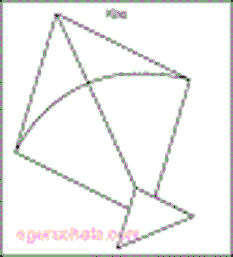### Kite Calculator

Calculator calculates the area of a kite with both methods (Trigonometry and Diagonal) and also calculate the perimeter of the kite.
Trigonometry method: Area = product of both side length sin (angle between them) = ab sin C, Where a and b are the lengths of two unequal sides, C is the angle
Diagonal method: Area = The area is half the product of the diagonals = (d1d2)/2 where d1,d2 are the length of diagonal.
Perimeter: add the length of each side = 2a+2b Where a and b are the lengths each sides.Area of Kite [Diagonal Method]: [ ½×d1d2 ] Enter the Diagonal lengths = Area of Kite =Area of Kite Using Trigonometry:[ ab Sin C ] Enter the lengths = Enter the angle = Area of Kite =Perimeter of Kite: [ 2(a+b) ] Enter the lengths = Perimeter of Kite =